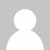Day 8’s challenge at Advent of Code was thankfully not as taxing as day 7. Here’s my video discussing how I solved it in C# and F#

My C# solution basically involved me doing the escaping and unescaping manually. As I mention in the video, it turned out to be a good thing, as the C# compiler’s rules for unescaping don’t match the challenge’s anyway.

``````File.ReadAllLines("day8.txt")
.Select(s => new
{
Escaped = s, Unescaped =
Regex.Replace(
s.Substring(1, s.Length - 2)
.Replace("\\\"", "\"")
.Replace("\\\\", "?"),
@"\\x[0-9a-f]{2}", "?")
})
.Sum(s => s.Escaped.Length - s.Unescaped.Length)
.Dump("a"); // 1333

.Select(s => new
{
Original = s,
Escaped = "\"" +
s.Replace("\\", "\\\\")
.Replace("\"", "\\\"") + "\""
})
.Dump("expanded")
.Sum(s => s.Escaped.Length - s.Original.Length)
.Dump("b"); // 2046
``````

For my F# version, I tried to clean the code up a little with some predefined constants as well as making one function that could solve both parts of the problem:

``````let q,b,bq,bb = "\"","\\","\\\"","\\\\"

let unescape (s:string)  =
Regex.Replace(s.Substring(1, s.Length - 2)
.Replace(bq, q)
.Replace(bb, "?"),
@"\\x[0-9a-f]{2}", "?")

let escape (s:string) = q + s.Replace(b, bb).Replace(q, bq) + q

let input = "day8.txt" |> File.ReadAllLines

let sumDiffLengths strings (f:string->string*string) =
strings
|> Seq.map f
|> Seq.sumBy (fun (a,b) -> a.Length - b.Length)

sumDiffLengths input (fun s -> (s, unescape s))
|> printfn "a: %d" // 1333

sumDiffLengths input (fun s -> (escape s, s))
|> printfn "b: %d" // 2046
``````
Want to learn more about LINQ? Be sure to check out my Pluralsight course LINQ Best Practices.``let common mapping =  System.IO.File.ReadAllLines "day08.txt"  |> Seq.sumBy (mapping >> (<||) (-))let part1 () =  let memoryLength =    let rec aux acc = function      '\\' :: ('\\' | '"') :: xs    | '\\' :: 'x' :: _ :: _ :: xs -> aux (acc + 1) xs    | '"' :: xs -> aux acc xs    | _ :: xs -> aux (acc + 1) xs    | [] -> acc  Seq.toList >> aux 0  common (fun str -> String.length str, memoryLength str)let part2 () = common (fun str -> 2 + Seq.sumBy (function '\\' | '"' -> 2 | _ -> 1) str, String.length str)/code>``